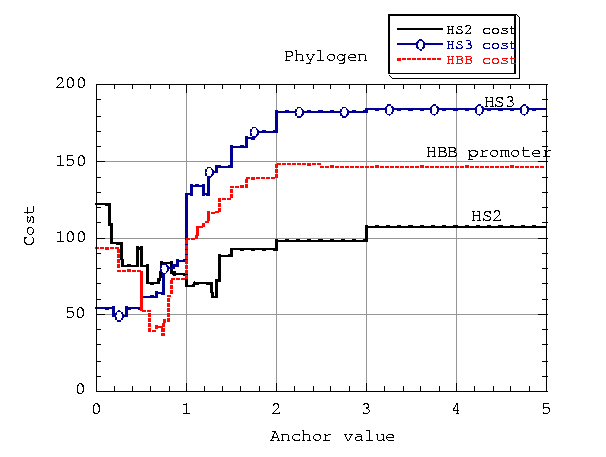## Discussion of calibration procedure

We conducted a series of tests on the multiple alignment of the beta-globin gene cluster (Hardison et al., 1997) using five utilities for finding conserved sequences (agree, infocon, phylogen, kkno, kunk) and varying the values of the relevant parameters for each method. The goal was to determine the sets of parameter values that would optimize (minimize) a chosen cost function. Specifically, the cost function considered in assessing the accuracy of the results was the total count of false positives and false negatives with respect to a set of landmarks. A false positive is a position in the human sequence which does not belong to any of the known functional sites, but was reported by the program under examination. A false negative is a position in the human sequence that belongs to a known functional site but was not reported by the program.

Two types of assessments were performed: per region assessments, targeted towards the HS2, HS3 and the HBB promoter regions individually, and overall assessments. In the latter case, the goal was to find the set of parameter values that would produce a best aggregate total count for the regions considered. We describe our methods next.

[kkno, kunk]
Parameters: k=1 fixed, l (required minimum region length).
Method:

• We tested kkno (kunk) for values of l=3..25 and recorded the number of false positives and false negatives, per region and overall.

[infocon]*
Parameters: l (required minimum region length), a (anchor value, or score adjustment factor).
Method:

• We tested infocon for values of l=3..25, varying a between -10.0 and 2.0, in increments of 0.001. As a became larger, the number of false positives decreased and the number of false negatives increased, as the regions obtained for larger a's were included in those obtained for smaller values. For each length l, we determined a partition of the interval [-10.0,2.0] of possible score adjustment values into sub-intervals for which the method produced stable results (i.e., the same number of false negatives and false positives, respectively, regardless of the a value chosen within the interval). Based on these data, we determined the best a-interval for every length l and the best overall pair of parameter values for a and l, according to the cost function proposed.

[phylogen]*
Parameters: l (required minimum region length), a (fixed anchor), mode (flexible anchor, if any).
Method:

• We tested phylogen for values of l=3..25, varying a, the number of false positives increasing and the number of false negatives decreasing as a became larger. Two sets of tests were performed: for mode=A, when the number of active rows in the column (flexible anchor) and the specified constant a (fixed anchor between -100.0 and 100.0) were both included in the score adjustment factor, and for mode=N, when no flexible factors were considered and the score was adjusted based solely on the value a, a constant between 0.0 and 100.0. As before, a partition of the interval of a-values was produced for each mode of operation, and the best a-intervals and best overall (a,l) pair were determined according to the cost criterion.

[agree]*
Parameters: l (required minimum region length), p (percent similarity threshold), mode (gap inclusive or not).
Method:

• We tested agree for values of length l=3..25, varying p between 10 and 100 in increments of 1%. The number of false positives and false negatives again varied monotonically with p, as the method achieved smaller coverage with increasing p values. Tests were performed separately for the gap inclusive (mode=G) and gap exclusive (mode=X) cases and results of the same form as for phylogen and infocon were obtained.

* NOTE
For all three of these programs (infocon, phylogen and agree), there is an efficient way to partition the interval of parameter values. Since the number of false positives and the number of false negatives vary monotonically with the value of the second parameter (a or p), the continuum of possible values can be explored using a binary-search-type procedure. This partitions the range of parameter values into intervals over which the program's results are invariant. For completeness, the intervals were chosen to cover more than the effective ranges, which were [ 0.0, 1.651064 ] for infocon, where 1.651064 is the maximum information content for the alignment tested, and [0.0,4.0] for phylogen (mode=N), since the maximum phylogenetic distance for a five-sequence alignment is 4. Intervals in the extremes of the parameter ranges gave identical costs, and thus fewer runs were made in those intervals, allowing these extreme intervals to be sampled at low computational cost. By this procedure, intervals in the more effective range were explored in smaller increments.

## Plot of the calibration curves for phylogenCalibration curve, showing the costs of results returned by phylogen while varying the anchor value. The anchor value was varied over the range from 0 to 5 in increments of 0.001, holding the minimum length l constant at the best value for each region. Each line has 5000 data points.